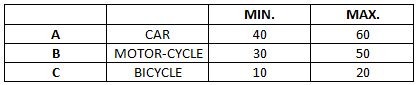### CAT 1997 Question Paper Question 32

Instructions

A certain race is made up of three stretches: A, B and C, each 2 km long, and to be covered by a certain mode of transport. The following table gives these modes of transport for the stretches, and the minimum and maximum possible speeds (in km/hr) over these stretches. The speed over a particular stretch is assumed to be constant. The previous record for the race is 10 min.Question 32

# Mr Hare completes the first stretch at the minimum speed and takes the same time for stretch B. He takes 50% more time than the previous record to complete the race. What is Mr Hare's speed for the stretch C?

Solution

Total time taken by Mr. Hare to complete the race = 50% more of (10 min.) = 15 min.= $$\frac{1}{4}$$ hr.
Or $$\frac{1}{4} = \frac{2}{40} + \frac{2}{40} + \frac{2}{v}$$ ( i.e. complete time for strech A,B and C Where v is velocity at strech C)
Or  v= 13.3 km./hr